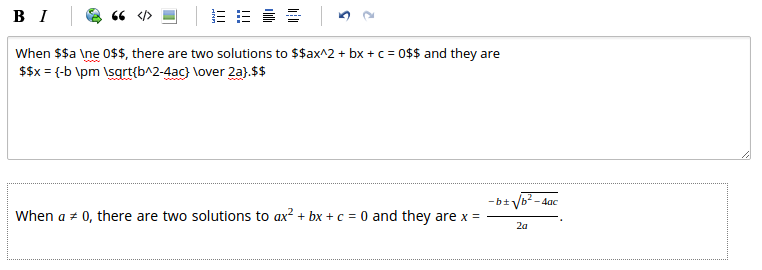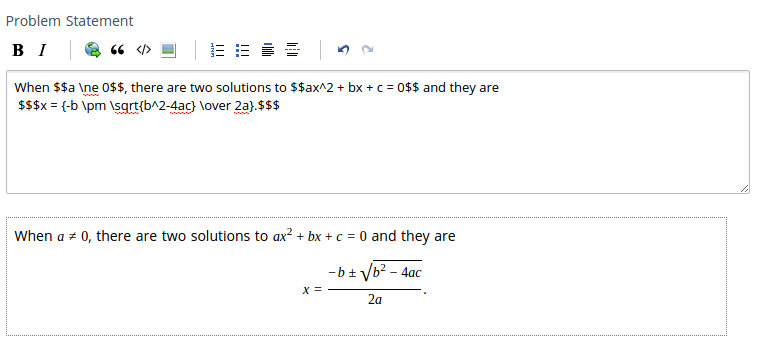# Math formatting guide

We now support latex throughout the site. We use Mathjax for rendering beautiful mathematical equations. You can create mathematical equations using tex macros.

### Usage:

To include math symbols in the content you need to use tex macros. Read more about tex here

Math symbols can be included in two ways, inline and new line.

To include math symbols in between words of a sentence instead of new line, inline LaTex is used. $is the delimiter for both opening and closing delimiters for newline LaTex. Check the example below.### Newline To include math symbols in new line, use newline latex.$\$ is the delimiter for both opening and closing delimiters for newline latex. Check the example below.Refer to wiki for complete list of latex symbols. MathJax library supports only math-mode macros. It does not support text-mode macros. Here is a list of macros MathJax supports.

Check this for good illustration of math-mode LaTeX commands.

Here is basic Mathjax guide.

Note:

For inline latex you need to put two backslashes before opening and closing brackets, because backslash is escape character in HackerEarth markdown editor. Hence first backslash is escaped.